Open in App
Not now

# Class 9 RD Sharma Solutions – Chapter 14 Quadrilaterals- Exercise 14.3

• Last Updated : 28 Mar, 2021

### Question 1. In a parallelogram ABCD, determine the sum of angles C and D.

Solution: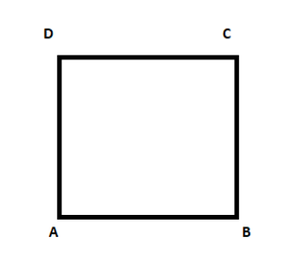In the given ||gm ABCD,

∠C + ∠D = 180°

Because∠C and ∠D are consecutive interior angles on the same side of CD traversal, so their sum is equal to 180°.

### Question 2. In a parallelogram ABCD, if ∠B = 135°, determine the measures of its other angles.

Solution: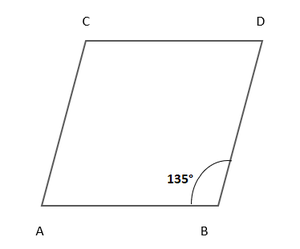In the given ||gm ABCD,

∠B = 135°

So ∠D = ∠B = 135° (Because opposite angles of a ABCD ||gm)

But ∠A + ∠B = 180° (Sum of consecutive angles) ….(i)

Now put the value of ∠B in eq(i)

∠B + 135° = 180°

∠A = 180° – 135° = 45°

But ∠C = ∠B = 45° (Opposite angles of a ||gm)

Here, the other angles are ∠A = 45°, ∠C = 45°, and ∠D = 135°.

### Question 3. ABCD is a square, AC and BD intersect at O. State the measure of ∠AOB.

Solution: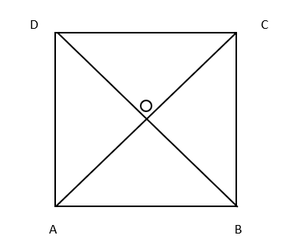In the given square ABCD,

Given that diagonal AC and BD intersect each other at O

Hence, ∠AOB = 90°, because in square diagonals are bisect each other at right angle.

### Question 4. ABCD is a rectangle with ∠ABD = 40°. Determine ∠DBC.

Solution: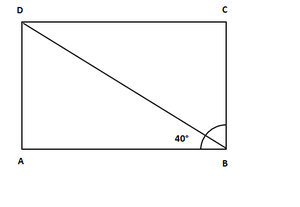In rectangle ABCD,

Given that ∠ABD = 40°, ∠B = 90°, BD is its diagonal

∠ABD + ∠DBC = 90° ……….(i)

Put the value of∠ABD = 40° in eq(i)

⇒ 40° + ∠DBC = 90°

⇒ ∠DBC = 90° – 40° = 50°

Hence, the value of angle ∠DBC = 50°

### Question 5. The sides AB and CD of a parallelogram ABCD are bisected at E and F. Prove that EBFD is a parallelogram.

Solution: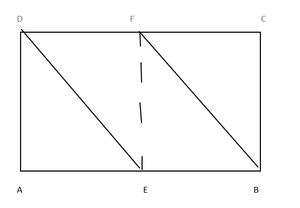In the given ||gm ABCD,

Given that E and F are the mid points of the line AB and CD

and DE and BF are joined

To prove: EBFD is a ||gm

Construction: Join EF

Proof: ABCD is a ||gm

So, AB = CD and AB || CD (Opposite sides of a ||gm are equal and parallel to each other)

EB || DF and EB = DF (Given that E and F are mid points of AB and CD)

Hence, proved EBFD is a ||gm.

### Question 6. P and Q are the points of trisection of the diagonal BD of the parallelogram ABCD. Prove that CQ is parallel to AP. Prove also that AC bisects PQ.

Solution: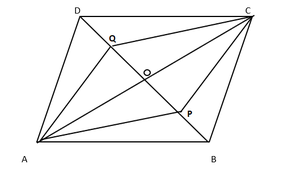In the given ||gm ABCD

It is given that P and Q are the points of trisection of the diagonal BD

To prove: (i) CQ || AP

(ii)AC bisects PQ

Proof: As we know that the diagonals of a parallelogram bisect each other

So, AO = OC and BO = OD

According to figure P and Q are point of trisection of BD

So BP = PQ = QD …(i)

BO = OD and BP = QD …(ii)

Now subtract, eq(ii) from eq(i) we get

OB – BP = OD – QD

⇒ OP = OQ

OA = OC and OP = OQ (proved above)

Diagonals AC and PQ bisect each other at O

So, APCQ is a parallelogram

Hence, AP || CQ

### Question 7. ABCD is a square. E, F, G, and H are points on AB, BC, CD, and DA respectively, such that AE = BF = CG = DH. Prove that EFGH is a square.

Solution: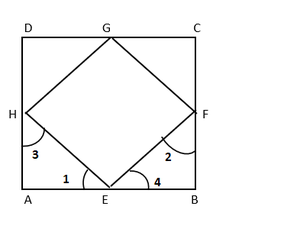In the given square ABCD

It is given that E, F, G and H are the points on AB, BC, CD and DA respectively

Such that AE = BF = CG = DH

To prove : EFGH is a square

Proof : It is given that E, F, G and H are points on the sides AB, BC, CA and DA respectively such that

AE = BF = CG = DH = x (suppose)

Then BE = CF = DG = AH = y (suppose)

Now in ∆AEH and ∆BFE

AE = BF (given)

∠A = ∠B (each 90°)

AH = BE (proved)

Hence, by SAS criterion

∆AEH ≅ ∆BFE

∴ ∠1 = ∠2 and ∠3 = ∠4 (C.P.C.T.)

But ∠1 + ∠3 = 90° and ∠2 + ∠4 = 90° (∠A = ∠B = 90°)

⇒ ∠1 + ∠2 + ∠3 + ∠4 = 90° + 90° = 180°

⇒ ∠1 + ∠4 + ∠1 + ∠4 = 180°

⇒ 2(∠1 + ∠4) = 180°

⇒ ∠1 + ∠4 = 180°/2 = 90°

∴ ∠HEF = 180° – 90° = 90°

Similarly, we can prove that

∠F = ∠G = ∠H = 90°

Since sides of the EFGH is are equal and each angle is of 90°

Hence, EFGH is a square.

### Question 8. ABCD is a rhombus, EABF is a straight line such that EA = AB = BF. Prove that ED and FC when produced meet at right angles.

Solution: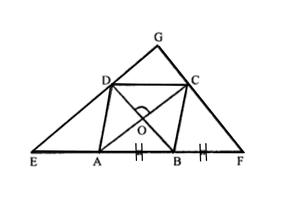Given that ABCD is a rhombus

EABF is a straight line such that

EA = AB = BF

Now join ED and FC

Which meet at point G

To prove: ∠EGF = 90°

Proof: As we know that the diagonals of a rhombus bisect

each other at right angles

AO = OC, BO = OD

So, ∠AOD = ∠COD = 90°

and ∠AOB = ∠BOC = 90°

In ∆BDE,

A and O are the mid-points of BE and BD respectively.

AO || ED

Similarly, OC || DG

In ∆ CFA, B and O are the mid-points of AF and AC respectively

OB || CF and OD || GC

Now in DOCG

OC || DG and OD || CG

Hence, DOCG is a parallelogram.

∠DGC = ∠DOC (Opposite angles of ||gm are equal)

∠DGC = 90° (because∠DOC = 90°)

Hence, proved

### Question 9. ABCD is a parallelogram, AD is produced to E so that DE = DC = AD and EC produced meets AB produced in F. Prove that BF = BC.

Solution: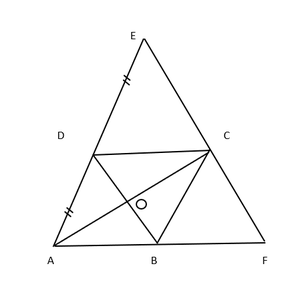In the given ||gm ABCD,

AB is produced to E so

Also EC produced meets AB produced in F.

To prove : BF = BC

Proof: In ∆ACE,

O and D are the mid points of sides AC and AE

DO || EC and DB || FC

⇒ BD || EF

AB = BF

But AB = DC (Opposite sides of ||gm are equal)

DC = BF

Now in ∆EDC and ∆CBF,

DC = BF (proved)

∠EDC = ∠CBF

(because ∠EDC = ∠DAB corresponding angles)

∠DAB = ∠CBF (corresponding angles)

∠ECD = ∠CFB (corresponding angles)

So, by ASA criterion,

∆EDC ≅ ∆CBF

DE = BC (By C.P.C.T.)

⇒ DC = BC

⇒ AB = BC

⇒ BF = BC (∵ AB = BF proved)

Hence, proved

My Personal Notes arrow_drop_up